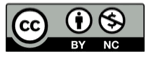# 内摆线

2018-06-05## R/r=2## R/r=3## R/r=4## R/r=5## R/r=5/3## 参数表达式

$$x(t)=(R-r) \cos (t) + r \cos ({{R-r} \over r}t)\\y(t)=(R-r) \sin (t) + r \sin ({{R-r} \over r}t)$$

## 精彩评论